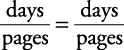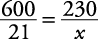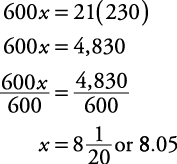## Ratio and Proportion

Here is an example of calculating ratios and proportions in word problems.

##### Example 1

If Arnold can type 600 pages of manuscript in 21 days, how many days will it take him to type 230 pages if he works at the same rate?

First, circle what you're asked to find— how many days. One simple way to work this problem is to set up a “framework” (proportion) using the categories given in the equation. Here the categories are pages and days.

Therefore, a framework may beNote that you also may have usedThe answer will be the same. Now simply plug into the equation for each instance.Cross multiplyingTherefore, it will takeor 8.05 days to type 230 pages. (You may have simplified the original proportion before solving.)